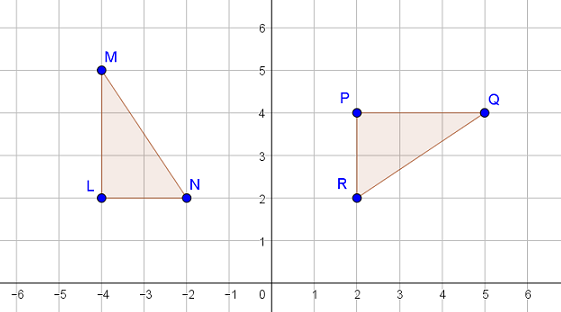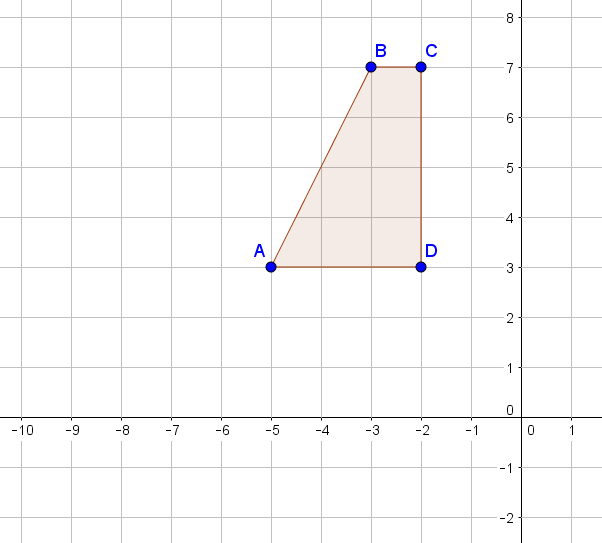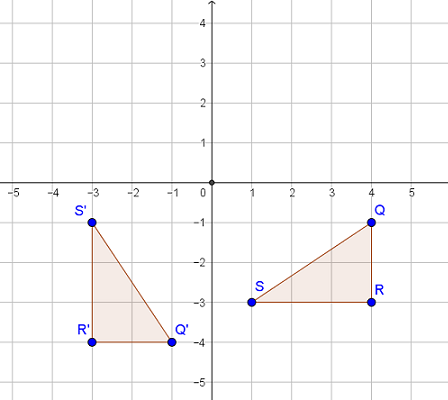# Transformations and Angle Relationships

## Objective

Describe and perform rotations between congruent figures.

## Common Core Standards

### Core Standards

?

• 8.G.A.1.A — Lines are taken to lines, and line segments to line segments of the same length.

• 8.G.A.1.B — Angles are taken to angles of the same measure.

• 8.G.A.1.C — Parallel lines are taken to parallel lines.

• 8.G.A.2 — Understand that a two-dimensional figure is congruent to another if the second can be obtained from the first by a sequence of rotations, reflections, and translations; given two congruent figures, describe a sequence that exhibits the congruence between them.

?

• 4.MD.C.6

## Criteria for Success

?

1. Describe rotations with a direction, an angle of rotation, and a point to rotate around.
2. Use patty (transparency) paper to rotate images.
3. Understand that in a rotation, every point on an original figure moves the same number of degrees and in the same direction around the same point to create the new figure.
4. Understand that rotations preserve shape, size, orientation, area, perimeter, and parallel line relationships, but they do not preserve location.

## Tips for Teachers

?

• This lesson focuses on rotations in isolation—how to do them and how to talk about them. Upcoming lessons will begin to combine rotations with the other rigid transformations.
• Similar to the video from Lesson 4, this video, Rotation, by PBS Learning Media introduces the concept of rotation and includes animation that can be helpful for students to visualize the movements, especially the importance of the point of rotation
• The following materials are useful for this lesson: patty paper (or transparency paper) and graph paper.

#### Fishtank Plus

• Problem Set
• Student Handout Editor
• Vocabulary Package

## Anchor Problems

?

### Problem 1

Triangle ${{LMN}}$ underwent a single transformation to become triangle ${{PQR}}$, shown below.1. What single transformation maps triangle ${{LMN}}$ to triangle ${{PQR}}$? Describe in detail.
2. Name two things that are the same about both triangles.
3. Name two things that are different about the triangles.

### Problem 2

Perform the following rotations on figure ${{ABCD}}$ below.

• Rotate figure ${{ABCD}}$  ${90^{\circ}}$ counter-clockwise around point $A$ to create $A'B'C'D'$.
• Rotate figure ${{ABCD}}$  ${180^{\circ}}$ counter-clockwise around point $A$ to create $A''B''C''D''$.## Problem Set

?The following resources include problems and activities aligned to the objective of the lesson that can be used to create your own problem set.

• Examples where students identify and describe a rotation between two figures (limit to around the origin and around a point on the figure only), similar to Anchor Problem #1
• Examples where students perform rotations around either the origin or a point on the figure, similar to Anchor Problem #2
• Examples where students identify new coordinate points of a figure after it is rotated
• Error analysis of figures that appear to be rotated but the orientation is shifted

?

Triangle ${QRS}$ was transformed to create triangle ${Q'R'S'}$ in the coordinate plane below.1. Describe the transformation that maps ${QRS}$ to ${Q'R'S'}$.
2. Rotate figure ${Q'R'S'}$ ${90^{\circ}}$ clockwise around the origin and draw the new figure ${{Q''R''S''}}$. Describe a single transformation that maps triangle ${QRS}$ to ${{Q''R''S''}}$.

?